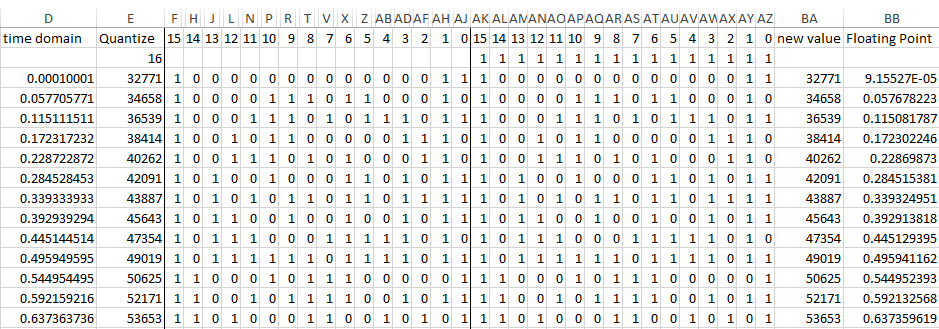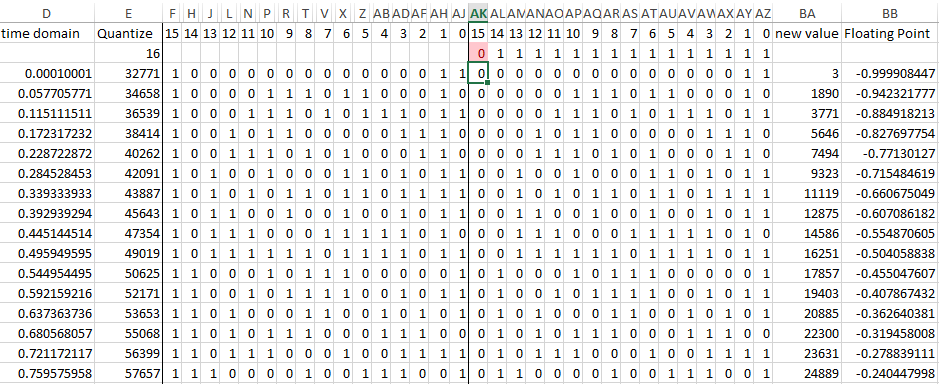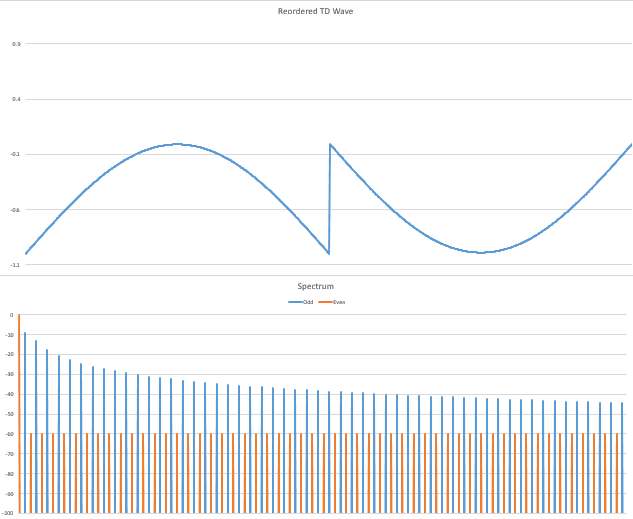Sine wave passed through an Excel quantizer

# DAC MSB fail simulation

Published on March 31, 2020

Knowing that some of my readers might be tired of my plain Jane distortion simulations, I decided to take a new tack, to use nautical jargon (as I live on a boat). So I decided the best way to do that was to simulate a bad bit going to a DAC (digital to analog converter for those of you in Rio Linda). So I made up a new Excel spreadsheet and spent a whole day putting together the quantizer, as Excel's DEC2BIN() function only works on values up to 512, and I needed to simulate something like a 16 bit DAC, so I had to craft my own. Then I added a mechanism to force one or more bits going to the DAC to zero throughout the whole wave, and then once again converted the value to a floating point number. In the image above you can see the value on the far left, 0.0001001, the first point in my 2048 point waveform. Converting to unsigned binary (with an offset of 32768) quantized to 16 bits is 32771, the value just to the right of that. Converted to binary, that is 1000 0000 0000 0011. Notice in the cells from F to AJ, every other column is missing. That's because I did the math inside hidden columns that you don't need to see, and neither do I. Let's just say it's magic. Now, moving over to the right in cells AK-AZ are 16 cells where the destination data goes, and above the data is a mask, currently set at 1111 1111 1111 1111, that is, all 16 bits will pass through, so you can see that the value 1000 0000 0000 0011. If the mask changes, the data in this field all the way down to the 2048th sample will lose data in that column. But for now, we needn't worry. So, in cell BA3 we see the original value, 32771, and then to the right of that we convert back to a floating point number so we can do the FFT, plotting, etc. But because of the quantization back on the far left, the new value is 9.15527e-5 or 0.0000915527, just a tiny fraction away from the original value of 0.0001001. All is well. If I do an FFT on this wave, it will be perfect. Trust me.

However, if I set any one of those bits in AK2-AZ2 to zero, it will kill all the bits below it, like this:Now you can see that bit 15 near the middle of the image is highlighted red because I made it zero, and that caused every 1 down the line, all the way down to sample 2048 to become a zero. Now, some were zero, but a large number of them were at a 1. Now look what it does to the values on the right. 32771, the first value in the Quantize column becomes 3. Gee, what's 32771 - 32678? Yep, that's 3 alright. And look at the impact that has on the Floating Point column! What was supposed to be a value close to zero is now -0.999908447. The same thing happens all the way down. So what should be a sine wave with no harmonics at all ends up looking like this:This is very bad! You can see what happened to the poor sine wave, and you can tell by the spectrum below it that there is a lot of DC in bin 0, as you would expect, since the entire wave is below zero. And while the time domain wave looks very symmetrical, there is a problem: We have Even harmonics. This doesn't make sense does it? In fact, the Even harmonics plow through the spectrum at the same amplitude all the way to the max frequency of the spectrum. But that doesn't make sense! Bullard Laws of Harmonics #3 says:

### "Even harmonics don't appear in symmetrical distortion because they cancel each other out."

So because the wave looks symmetrical, it shouldn't have any Even harmonics. But, looks can be deceiving. How do we figure out what is asymmetrical about this wave? This question bothered me for a day, the next morning I had a solution. I would take the complex data from the FFT of the wave, zero out all the Even harmonics and then do an inverse FFT and compare the two Time Domain waves, as I show them here:In this plot, the blue wave is the one with no Even harmonics. Notice in the wide shot (above) that the only time the blue wave is different from the red (the one with Even harmonics) wave is at the very beginning where it starts at -0.5 and then drops down. That confused me for a while. But then I looked at the center of the wave (lower plot) and saw what was going on. By removing the Even harmonics, I forced the wave to be symmetrical in time. That's what happened to the wave when I removed the MSB, it remained symmetrical in voltage, but it became asymmetrical in time. Once I removed the Even harmonics, the fast rising edge that was caused by removing the MSB happened too early, and made the wave asymmetrical in time, but when I removed the Even harmonics and did the inverse FFT, it forced the time domain wave to become symmetrical in time, preserving Bullard Laws of Harmonics #3.

So my laws do hold up under the craziest tests you can come up with. The one thing to remember here is that it would be damn hard to figure this out if I hadn't already discovered Law #3. My books and all my previous articles gave you boring, but real life examples. But straying off the reservation can lead to insights too, but there is a reason that nobody else ever figured out how harmonics come about, and this example proves that to us once again.